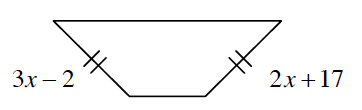### Home > INT2 > Chapter 7 > Lesson 7.2.2 > Problem7-86

7-86.

Multiple Choice: Which equation below correctly represents the relationship of the side lengths of the trapezoid given in the diagram below?1. $3x - 2 + 2x + 17 = 360^\circ$

1. $3x - 2 + 2x + 17 = 180^\circ$

1. $3x - 2 + 2x + 17 = 90^\circ$

1. $3x - 2 = 2x + 17$

If the angles have the same measures, what does that tell you about the legs of the trapezoid?

D